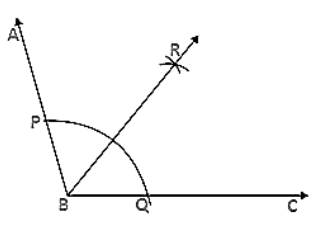# Draw an obtuse angle.

Question:

Draw an obtuse angle. Bisect it. Measure each of the angles so formed.

Solution:

Steps of construction:

1. Draw an angle ∠ABC of 120°.

2. With B as a centre and any radius, draw an arc which intersects AB at P and BC at Q.

3. With P as center and radius more than half of PQ draw an arc.

4. With Q as a center and same radius draw an arc which cuts the previous arc at R.

5. Join BR.

Therefore ∠ABR = ∠RBC = 60°